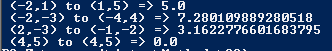# Assignemnt #93 and Distance Formula

## Code

```
/// Name: David Shkolnikov
/// Period: 6
/// Program Name: Distance Formula
/// File Name: DistanceFormula.java
/// Date Finished: 3/21/2016

public class DistanceFormula
{
public static void main( String[] args )
{
// test the formula a bit
double d1 = distance(-2,1 , 1,5);
System.out.println(" (-2,1) to (1,5) => " + d1 );

double d2 = distance(-2,-3 , -4,4);
System.out.println(" (-2,-3) to (-4,4) => " + d2 );

System.out.println(" (2,-3) to (-1,-2) => " + distance(2,-3,-1,-2) );

System.out.println(" (4,5) to (4,5) => " + distance(4,5,4,5) );
}

public static double distance( int x1, int y1, int x2, int y2 )
{
double a, b, D;

a = x2 - x1;
b = y2 - y1;
D = Math.sqrt( a*a + b*b );

return D;
}
}

```

### Picture of the output